Next: On "confirmation" of the SRT conservation laws Up: Dynamics of the SRT Previous: Additional remarks   Contents

# Criticism of the conventional interpretation of relativistic dynamics

As a preliminary, to avoid a series of misunderstandings some comments should be made in respect to relativistic mechanics. First, confirmation within experimental accuracy for the laws of motion (the observable net results) cannot be considered as justification of all the methods used to obtain these results. In a scientific theory net results as well as starting principles and intermediate methods must all themselves be true as such! Second, arguing SRT's basic notions of space and time to be erroneous in no way implies a return to classical mechanics with static forces for the description of real particle motions. These two theories are not interrelated in any way. Classical mechanics is a model theory; it assumes bodies to be absolutely solid, impact of two material points (actually - two absolutely solid elastic spheres, whose radii tend to zero in the limit) to be absolutely elastic; kinetic energy and momentum to be fully "concentrated" in the motion of a body as a whole, and the exchange of energy and momentum to occur instantaneously. Neither classical mechanics nor relativity theory investigates the processes inside colliding particles; the only additional question about the rate of transmission of interactions appears at high velocities (about accounting finiteness of this rate).

Of course, taking account of a finite time for propagation and transmission of interactions results in a change of the observed motion of particles. An additional dependence of quantities on velocity appears; for example, in an effective mass (more precisely for the effective force). This can be understood qualitatively from the following elementary mechanical model: Consider just one-dimensional motion; let a source emit continuously and uniformly similar particles flying at some constant speedalong the straight line. At any place of the straight line, a sample body put to rest will undergo action of a constant pressure force (from bombarding particles). If we now put a sample body moving away from the source at some velocity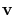, then the number of particles reaching this body per time unit will decrease. This can be interpreted as a decrease of the effective force, or increase of the effective mass. In the limit,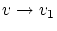the effective mass of the body being accelerated under the effect of particles tends to infinity (more correctly to say, the effective force tends to zero).

Certainly, it is impossible to deduce quantitative dependences from this classical mechanistic model, because the collisions themselves cannot be considered as absolutely elastic and instantaneous. Recall only that there exists the classical Lorentz model (a deformable sphere), which describes the dynamics of an electron (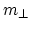and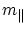). The classical equation of motion for particles can also be obtained considering non-locality or non-linearity [14,15,81]. Relativistic effects can be also obtained with assuming change of the effective charge. The aim of this book does not include the analysis of all possible methods of development of relativistic mechanics, or the choice between these methods.

Now we shall directly proceed to the relativistic dynamics. SRT is completely inconsistent in considering accelerations and the dynamics of particles in general. The Lorentz transformations (from which the entire SRT issues) cannot impose any limitations on accelerations of bodies (as well as on studying accelerated systems). However, in such a case some SRT mismatches with the experiment would become too noticeable. As a result, SRT artificially declares that the study of accelerated (non-inertial) systems is a prerogative of GRT. But the successive application of this declaration would remain from SRT only the Lorentz transformations themselves and the velocity addition law (that is, a part of kinematics). To rise the "significance" of the theory, at first, in SRT the 4-acceleration is calculated formally mathematically, and then the relativistic dynamic equations are formally "derived". But what about the transformation of forces? In this case, contrary to SRT's own declaration, it is necessary to transform one accelerated particle (for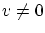) into "another" accelerated particle (for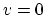). The transformation of electromagnetic fields also contradicts the declared self-limitations, since the fields, introduced in a conventional manner, reflect nothing but the action of electromagnetic forces (the force approach). It would seem that the declaring of equivalence of SRT and GRT approaches could rise the "significance" of the theory. However, in some problems the application of SRT and GRT leads to different quantitative results. These mismatches result in the necessity of sacrificing any of the relativistic theories (or, more correctly, both of them).

SubsectionsNext: On "confirmation" of the SRT conservation laws Up: Dynamics of the SRT Previous: Additional remarks   Contents
Sergey N. Arteha